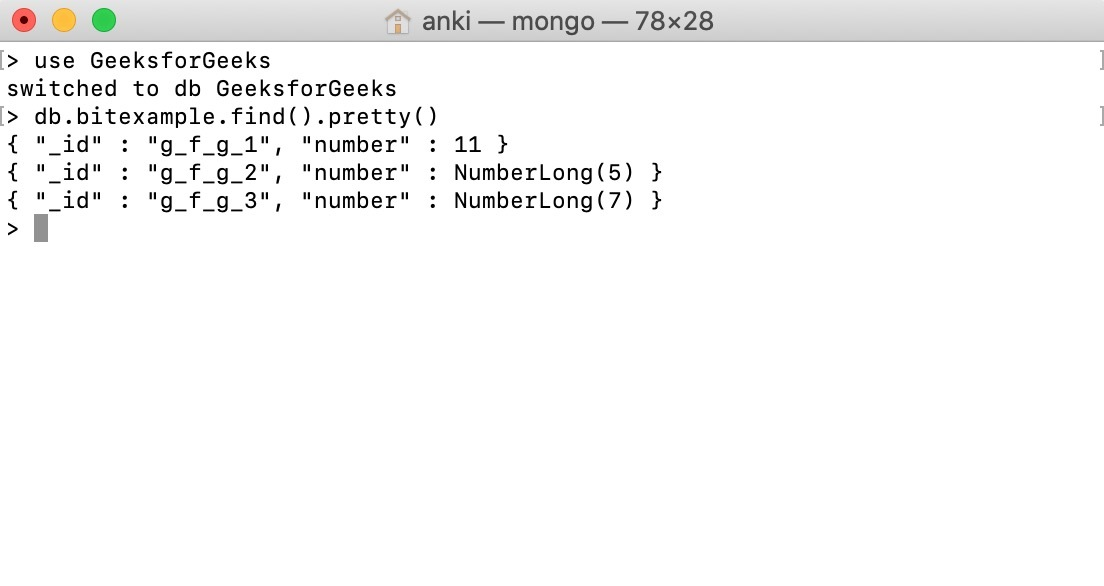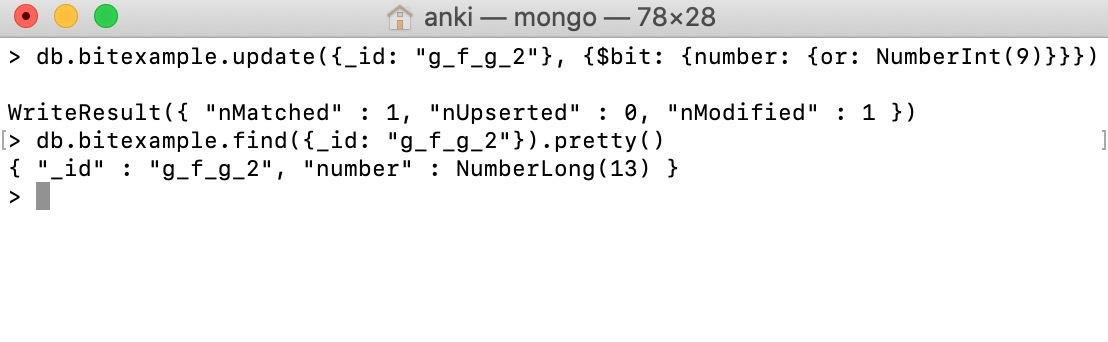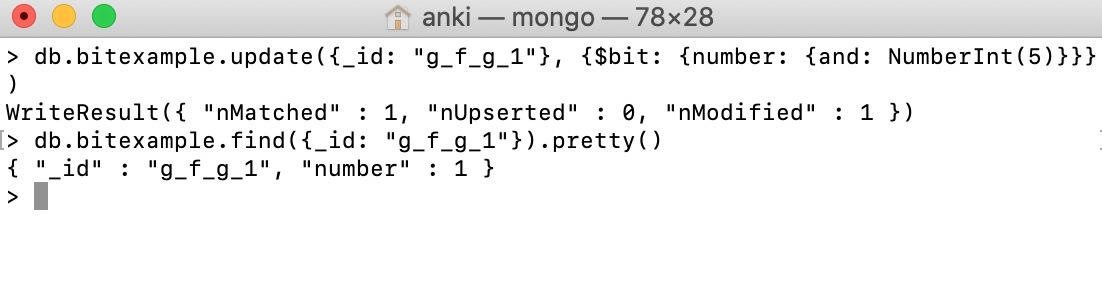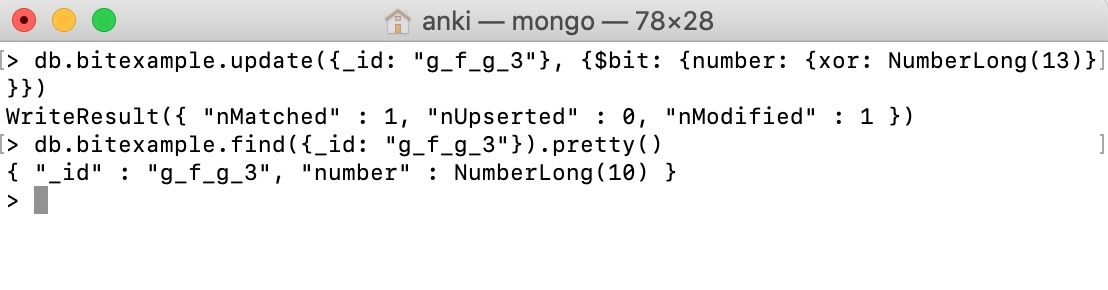# MongoDB Bitwise Update Operator

• Last Updated : 28 Jul, 2020

MongoDB provides a \$bit operator to perform a bitwise update of a field. This operator supports bitwise xor, bitwise or, and bitwise and operations.

Syntax:

```{ \$bit: { <field1>: { <or|and|xor>: <int> } } }
```

Important Points:

• Use \$bit operator only with integer fields(either 32-bit integer or 64-bit integer)
• To specify a field in embedded/nested documents or in an array use dot notation.
• All the numbers in the mongo shell are double not an integer. So, you need to use NumberInt() or the NumberLong() constructor to specify integers.
• You can use this operator in methods like update(), findAndModify(), etc., according to your requirements.

In the following examples, we are working with:

Database: GeeksforGeeks
Collection: bitexample
Document: three documents that contain the data in the form of field-value pairs.## Bitwise OR:

In this example, we are using update() method to update the value of number field of the document(id: g_f_g_2) to the result(i.e. NumberLong(13) or 1101) of a bitwise or operation perform between the current value NumberLong(5) (i.e. 0101) and NumberInt(9) (i.e. 1001):

```db.bitexample.update({_id: "g_f_g_2"},
{\$bit: {number: {or: NumberInt(9)}}})
```## Bitwise AND:

In this example, we are using update() method to update the value of number field of the document(id: g_f_g_1) to the result(i.e. NumberInt(1) or 0001) of a bitwise and operation perform between the current value NumberInt(11) (i.e. 1011) and NumberInt(5) (i.e. 0101):

```db.bitexample.update({_id: "g_f_g_1"},
{\$bit: {number: {and: NumberInt(5)}}})
```## Bitwise XOR:

In this example, we are using update() method to update the value of number field of the document(id: g_f_g_3) to the result(i.e. NumberLong(10) or 1010) of a bitwise xor operation perform between the current value NumberLong(7) (i.e. 0111) and NumberLong(13) (i.e. 1101):

```db.bitexample.update({_id: "g_f_g_3"},
{\$bit: {number: {xor: NumberLong(13)}}})
```My Personal Notes arrow_drop_up